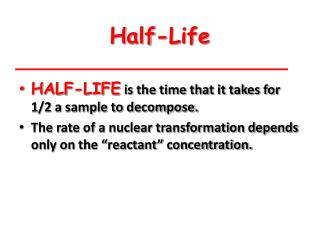DownloadDownload PresentationHalf-Life

# Half-Life

Télécharger la présentation## Half-Life

- - - - - - - - - - - - - - - - - - - - - - - - - - - E N D - - - - - - - - - - - - - - - - - - - - - - - - - - -
##### Presentation Transcript

1. Half-Life • HALF-LIFE is the time that it takes for 1/2 a sample to decompose. • The rate of a nuclear transformation depends only on the “reactant” concentration.

2. Half-Life Decay of 20.0 mg of 15O. What remains after 3 half-lives? After 5 half-lives?

3. Kinetics of Radioactive Decay For each duration (half-life), one half of the substance decomposes. For example: Ra-234 has a half-life of 3.6 daysIf you start with 50 grams of Ra-234 After 3.6 days > 25 grams After 7.2 days > 12.5 grams After 10.8 days > 6.25 grams

4. A daughter DN rate = - Dt Kinetics of Radioactive Decay N = N0(1/2)t/t1/2 lnNt= lnN0- kt N = the # of atoms at time t N0= the # of atoms at time t = 0 k is the decay constant (sometimes called l)

5. A radioactive substance has a half-life of 20 minutes. If we begin with a 500 g sample, how much of the original sample remains after two hours? Guestimation method Make a table with 2 columns: 1st column time elapsed, 2nd column mass of isotope

6. Now fill the first column with time increasing by half-life intervals Until you reach the end time mentioned in the question.

7. Now half the mass every time interval This shows how much mass would be left after each ½ life, so after 2 Hrs. 7.8125 grams would be left

8. The half-life of a material is 2 hours.If the initial count rate is 864 Bq,how long will it take for the count rate to fall to 27 Bq

9. The half-life of a material is 3 hours. If the initial count rate is 544 Bq, what will the count rate be after 15 hours? Guestimation method